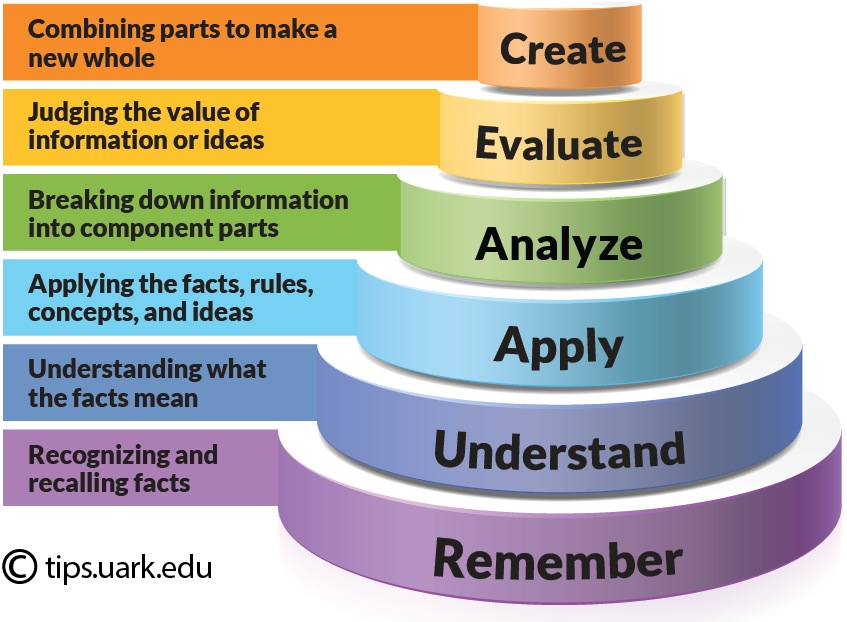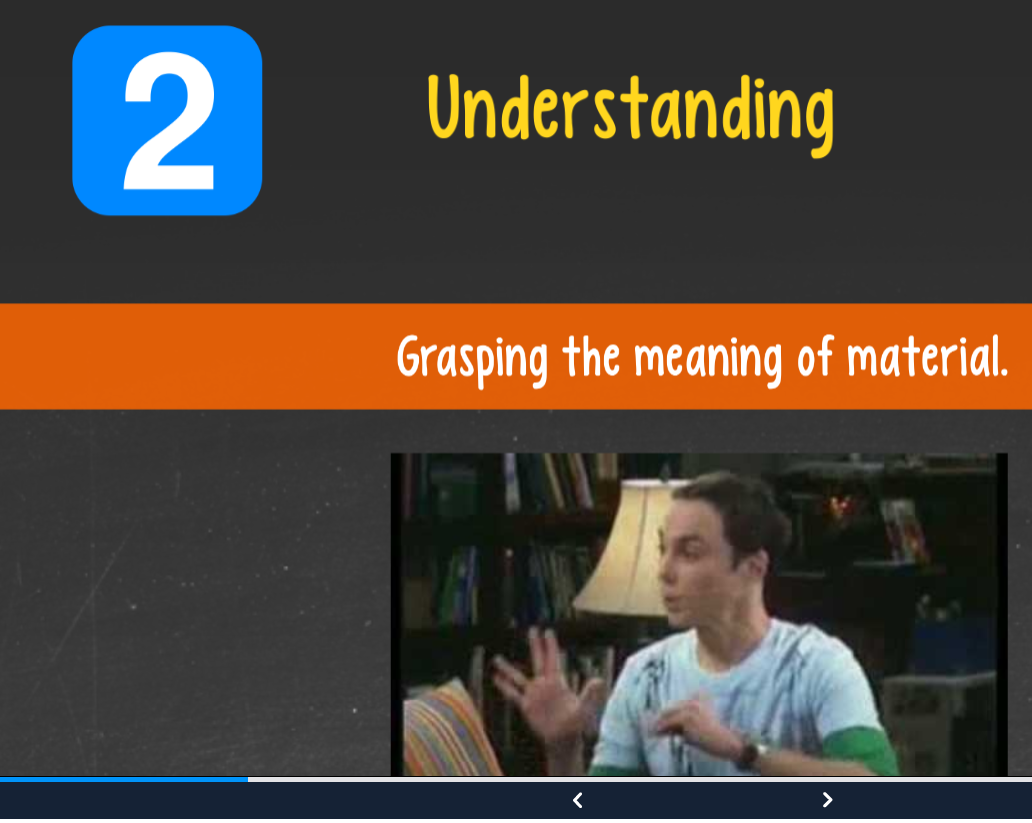## Bloom’s Learning Taxonomies

One way to approach learning objectives is through Bloom’s Learning Taxonomies.Bloom’s taxonomy is a powerful tool to help develop learning objectives because it explains the process of learning:

• Before you can understand a concept, you must remember it.
• To apply a concept you must first understand it.
• In order to evaluate a process, you must have analyzed it.
• To create an accurate conclusion, you must have completed a thorough evaluation.

However, we don’t always start with lower order skills and step all the way through the entire taxonomy for each concept you present in your course. That approach would become tedious–for both you and your students! Instead, start by considering the level of learners in your course.

Bloom’s LevelKey Verbs (keywords)Example Learning Objective
Createdesign, formulate, build, invent, create, compose, generate, derive, modify, develop.By the end of this lesson, the student will be able to design an original homework problem dealing with the principle of conservation of energy.
Evaluatechoose, support, relate, determine, defend, judge, grade, compare, contrast, argue, justify, support, convince, select, evaluate.By the end of this lesson, the student will be able to determine whether using conservation of energy or conservation of momentum would be more appropriate for solving a dynamics problem.
Analyzeclassify, break down, categorize, analyze, diagram, illustrate, criticize, simplify, associate.By the end of this lesson, the student will be able to differentiate between potential and kinetic energy.
Applycalculate, predict, apply, solve, illustrate, use, demonstrate, determine, model, perform, present.By the end of this lesson, the student will be able to calculate the kinetic energy of a projectile.
Understanddescribe, explain, paraphrase, restate, give original examples of, summarize, contrast, interpret, discuss.By the end of this lesson, the student will be able to describe Newton’s three laws of motion to in her/his own words
Rememberlist, recite, outline, define, name, match, quote, recall, identify, label, recognize.By the end of this lesson, the student will be able to recite Newton’s three laws of motion
Learning objective examples adapted from, Nelson Baker at Georgia Tech: nelson.baker@pe.gatech.edu